AP State Board Syllabus AP SSC 10th Class Physics Solutions Chapter 1 Heat Textbook Questions and Answers.

## AP State Syllabus SSC 10th Class Physics Solutions 1st Lesson Heat

### 10th Class Physics 1st Lesson Heat Textbook Questions and Answers

Question 1.
What would be the final temperature of a mixture of 50 g of water at 20° C temperature and 50 g of water at 40° C temperature? (AS1)
In CGS system :
Mass m1 = 50 g
Higher temperature = T1 = 40° C
Mass m2 = 50 g
Lower temperature = T2 = 20° C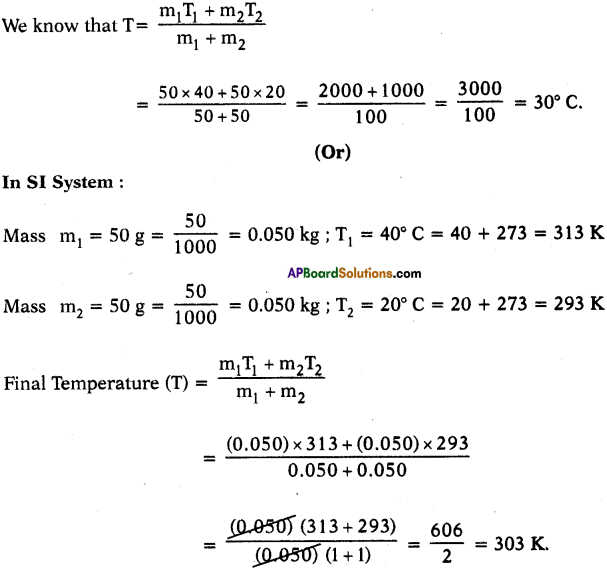Question 2.
Explain, why dogs pant during hot summer days using the concept of evaporation. (AS1)
(OR)
How do dogs cool their body? Explain by using the process of evaporation.

• Dogs pant during hot summer days and get their body cooled. This cooling effect is due to evaporation.
• Evaporation is a surface phenomenon. Temperature of a system falls during evaporation.
• During summer the temperature in the human body increases.
• The temperature of the skin becomes higher and the water in the sweat glands starts evaporating. Since evaporation is a cooling process human body becomes cool.
• Dogs don’t have sweat glands. Their body is covered with hair. They have sweat glands only in their feet.
• So by panting the water on the tongue undergoes evaporation resulting in the cooling of the dog’s body.

Question 3.
Why do we get dew on the surface of a cold soft drink bottle kept in open air? (AS1)
(OR)
Raju observed small droplets of water outside a cold soft drink bottle kept in open air. What is the reason for the formation of droplets?

• When cold soft drink bottle is kept in open air, the temperature of surrounding air is higher than the temperature of cold drink bottle.
• Air contains molecules in the form of vapour.
• During the motion of water molecules in air strike the surface of cold drink bottle.
• Then the molecules of air lose their kinetic energy which leads to lower the temperature and they convert into droplets.
• So dew is formed on the surface of cold soft drink bottle.Question 4.
Write the differences between evaporation and boiling. (AS1)
(OR)
Sita observed decrease in quantity of spirit kept in a vessel placed in open air. Whereas Ramu observed formation of bubbles on a water surface when it is heated. What are those two processes? Distinguish between those two processes.

 Evaporation Boiling 1. The process of escaping of molecules from the surface of a liquid at any temperature is called evaporation. 1. The process in which the liquid phase changes to gaseous phase at constant temperature. This temperature is called boiling point of liquid. 2. Evaporation takes place at any temperature. 2. Boiling takes place at a definite temperature. 3. The temperature of liquid gets down. 3. The temperature of liquids increases up to a constant temperature. 4. The kinetic energy does not change. 4. The kinetic energy of the molecules increases with the increase of temperature. 5. The evaporation depends on surface area, wind, speed, humidity. 5. The boiling depends on atmospheric pressure. 6. It is surface phenomenon. 6. It is bulk phenomenon. 7. Eg : 1) Wet clothes dries. 2) Sea water evaporates to form clouds. 7. Eg : 1) Water boils at 100° C.

Question 5.
Does the surrounding air become warm or cool when vapour phase of H2O condenses? Explain. (AS1)

• Gases have more higher energy than liquids and solids.
• When vapour condenses, it changes from gas to liquid.
• Therefore there is a drop in energy.
• This energy has to go (somewhere) to the surroundings.
• So surrounding air becomes warm when vapour phase of H20 condenses.

Question 6.
a) How much energy is transferred when 1 gm of boiling water at 100°C condenses to water at 100°C?
CGS system :
Mass of water = m = 1 gm
Latent heat of vapourisation = 540 cal/gm.
The amount of heat energy released when 1 gm of boiling water at 100°C condenses to water at 100°C
Q = mLvapour = 1 × 540 = 540 cal.

(OR)

In SI system :
Mass of water = m = 1 gm = 1 × 10-3 kg
Latent heat of vapourisation = 540 cal/gm.
The amount of heat energy released when 1 gm of boiling water at 100°C condenses to water at 100°C
Q = mLvapour – 1 × 540 = 540 cal.
In SI, Q = 540 × 4.18 = 2257 J.

b) How much energy is transferred when 1 gm of boiling water at 100° C cools to water 0° C?
CGS system :
Latent heat of vapourisation = 540 cal/gm
The amount of heat energy released when 1 gm of boiling water at 100°C condenses to water at 100 °C. m = 1 gm.
Q1 = mLvapour = 1 × 540 = 540 cal.
The specific heat of water = 1 cal/gm-°C
Difference in temperature = 100-0 = 100°C.
The heat released to cool water to 0°C is
Q2 = mS∆T = 1 × 1 × 100 = 100 cal.
∴ Total energy released = 540 + 100 = 640 cal.

c) How much energy is released or absorbed when 1 gm of water at 0° C freezes to ice at 0° C?
In CGS system :
Mass of water = m = 1 gm
Latent heat of fusion of ice (L) = 80 cal/gm
The energy transferred or released when 1 gm of water at 0° C freezes to ice at 0° C.
Q = mLfreeze = 1 × 80 = 80 Cal.

(OR)

In SI system :
Mass of water = m = 1 gm = $$\frac{1}{1000} \mathrm{~kg}$$
Latent heat of fusion = L = 3.36 × 105 J/kg.
Amount of heat released or transferred when lgm of water at 0°C freezes to ice at 0°C.
Q = mLfusion = $$\frac{1}{1000} \mathrm{~kg}$$ × 3.36 × 105 = 3.36 × 102 = 336J.

(OR)

In CGS system :
Mass of water = m = 1 gm
Latent heat of fusion of ice (L) = 80 cal/gm
The energy transferred or released when 1 gm of water at 0° C freezes to ice at 0°C.
Q = mLfreeze = 1 × 80 – 80 cal.
(Or)
In SI system : In SI, Q = 80 × 4.2 [1 cal = 4.2 J]
Q = 1 x 10-3 × 3.36 × 105 = 3 36 J.

d) How much energy is released or absorbed when 1 gm of steam at 100°C turns to ice at 0°C?
In CGS system :
Mass of water = m = 1 gm
Latent heat of vapourisation = Lvapour = 540 cal/gm
Latent heat of fusion of ice = Lfusion = 80 cal
Specific heat of water = S = 1 cal/gm-0°C
Difference in temperature
∆T = 100 – 0 = 100°C.
The energy transferred when 1 gram of steam at 100°C turns to ice at 0°C
Q = mLvapour + mS∆T + mLfusion
= 1 × 540 + 1 × 1 × 100 + 1 × 80 = 540 + 100 + 80 = 720 cal.

(OR)

Mass of water = m = 1 gm = $$\frac{1}{1000} \mathrm{~kg}$$
Latent heat of vapourisation = Lvapour = 2.25 × 106 J/kg
Latent heat of fusion = Lfusion = 3.36 × 105 J/kg
Difference in temperature
∆T = 373 – 273 – 100 K.
Specific heat of water = 4180 J/kg-K
The energy transferred when 1 gram of steam at 100° C turns to ice at 0°C =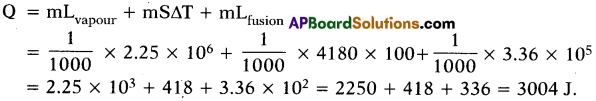(OR)

In CGS system :
Conversion : Steam at 100°C → Water at 100°C → Water at 0°C → Ice at 0°C.
Mass of water = m = 1 gm
Latent heat of vapourisation = Lvapour = 540 cal/gm
Latent heat of fusion of ice = Lfusion = 80 cal
Specific heat of water = S = 1 cal/gm-0°C
Difference in temperature
∆T= 100-0 = 100°C.
The energy transferred when 1 gram of steam at 100°C turns to ice at 0°C
Q = mLvapour + mS∆T + mLfusion
= 1 × 540 + 1 × 1 × 100 + 1 × 80
= 540 + 100 + 80 = 720 cal. = 720 × 4.18 = 3009.6 J.Question 7.
Explain the procedure of finding specific heat of solid experimentally. (AS1)
(OR)
Determination of specific heat of solid experimentally.
(OR)
Ravi wanted to prepare solid with high specific heat to use on cooking utensil. What fool does he need to find the specific heat of aluminium and copper? How should he conduct the experiment?
Aim : To find the specific heat of given solid.
Apparatus : Calorimeter, thermometer, stirrer, water, steam heater, wooden box and lead shots.

Procedure:

• Measure the mass of the calorimeter with stirrer = m1 gm
• Fill water one third volume of calorimeter and measure the mass = m2 gm.
• At this time initial temperature = T1.
• Mass of the water = m2 – m1 gm.
• Take a few lead shots and place them in steam heater and heat up to 100° C. Let this temperature be T2.
• Transfer the lead shots into calorimeter and measure the final (or) resultant temperature T3.
• Mass of calorimeter with contents = m3 gm and mass of lead shots = m3 – m2 gm.
• If the specific heats of the calorimeter, lead shots and water are Sc, Sl and Sw respectively, by using method of mixtures we have
Heat lost by the solid = Heat gained by the calorimeter + water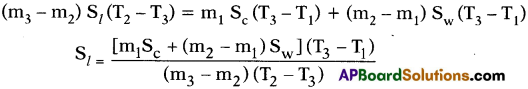• Knowing the specific heats of calorimeter and water we can calculate specific heat of solid (lead shots).

Question 8.
Convert 20° C into Kelvin scale. (or) Change 20°C into absolute scale. (AS1)
T = t°C + 273 = 20 + 273 = 293
⇒ T = 293 K.

Question 9.
Your friend is asked to differentiate between evaporation and boiling. What questions could you ask to make him to know the differences between evaporation and boiling? (AS2)
(OR)
Veena found that the water kept in a pot is cool and Siva observed when water is heated the temperature remains constant for some time until water turns into vapour. What are the processes involved in these two aspects? Ask some questions to understand these aspects.
The questions asked by me are :

• How do wet clothes get dried without heating?
• Are boiling and evaporation one and same or different?
• Is there any difference in kinetic energy if it boils?
• Is the temperature the main cause for boiling and evaporation?
• What are the factors which influence evaporation?
• Is boiling temperature for water always 100° C?Question 10.
What happens to the water when wet clothes dry? (AS3)

1. When wet clothes dry, the water present in the clothes is evaporated.
2. So that the process of evaporation causes the wet clothes dry.

Question 11.
Equal amounts of water are kept in a cap and in a dish. Which will evaporate faster? Why? (AS3)
(OR)
Srinu kept. equal amounts of water in a cap and in a dish in open air. What is his observation? Explain the experiment.
Aim : To show the evaporation of equal amounts of water in cap and dish.
Apparatus : Cap, dish, water.

Procedure :

• Take equal amounts of water in cap and dish. Keep them in open air for two hours. Now weigh the water in the cap and the dish.
• We can observe that the weight of water in dish is less than that of water in cap.
• This shows that the water in dish has more evaporation than the water in cap.
• It is due to more surface area of dish.
• As the surface area increases rate of evaporation also increases.

Question 12.
Suggest an experiment to prove that rate of evaporation of a liquid depends on its surface area and vapour already present in surrounding air. (AS3)
Aim: The rate of evaporation of liquid depends on its surface area and vapour already present in surrounding air.
Apparatus : Two dishes of different surface areas and water.

Procedure :

• Take two dishes of different surface area.
• Pour equal amounts of water in the both dishes.
• Keep aside for two to three hours.
• Observe them after some time.
Dish with more surface area has less quantity of water than the dish having less surface area. ,
• This shows evaporation increases with increasing of surface area.
• Take two dishes of equal surface area containing water.
• This experiment should be conducted on more humid day and less humid day.
• We will find that evaporation is less on more humid day due to more vapour in the air.
• So evaporation decreases with vapour in the air.

Question 13.
Place a Pyrex funnel with its mouth-down in a sauce pan full of water, in such a way that the stem tube of the funnel is above the water or pointing upward into air. Rest the edge of the bottom portion of the funnel on a nail or on a coin so that water can get under it. Place the pan on a stove and heat it till it begins to boil. Where do the bubbles form first? Why? Can you explain how a natural geyser works using this experience? (AS4)

• When Pyrex funnel with its mouth down in a sauce pan then the bubbles formed by the heat energy come from the top of the funnel.
• That is from stem tube.
• This is because of pressure inside mouth of funnel increases rapidly due to increasing of heat energy.
• Pressure inside the funnel rs more than outside the funnel and very high at stem.
• Hence, bubbles come from stem of the funnel and escapes through stem tube with force, like a geyser.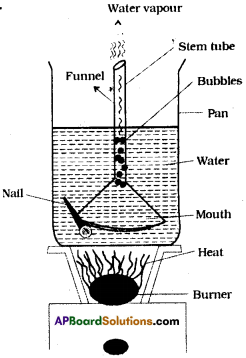Working of natural geyser by using this experience :

• Geysers are the fountains of hot water coming under the layers of the earth.
• It is a hole with narrow and deep from the bottom of the earth layers.
• It contains water.
• Water heats up due to high temperatures of the inner layers of the earth.
• As by the pressure of water at top layers of the hole, temperature rises, water boils.
• This hot water comes with narrow vent with high pressure, like Lava from the Volcano.

Question 14.
Collect the information about working of natural geyser and prepare a report. (AS4)
Natural Geysers :

• Geysers are the fountains of hot water coming under the layers of the earth.
• It is a hole with narrow and deep from the bottom of the earth layers.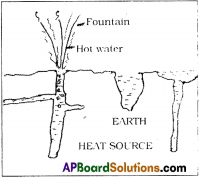• It contains water.
• Water heats up due to high temperatures of the inner layers of the earth.
• As by the pressure of water at top layers of the hole, temperature rises, water boils.
• This hot water comes with narrow vent with high pressure, like Lava from the Volcano.
• This looks like a water fountain at the surface of the earth.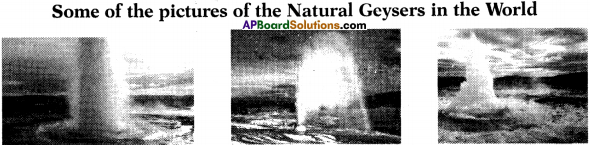Question 15.
Assume that heat is being supplied continuously to 2 kg of ice at – 5°C. You know that ice melts at 0°C and boils at 100°C. Continue the heating till it starts boiling. Note the temperature for every minute. Draw a graph between temperature and time using the values you get. What do you understand from the graph ? Write the conclusions. (AS5)
Graph between time and temperature from ice melting at 5° C to boils at 100° C.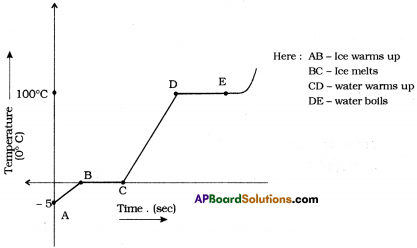Understanding from the graph :

• $$\overline{\mathrm{AB}}$$ = Ice warms up from – 5°C to 0°C
• $$\overline{\mathrm{BC}}$$ = Ice melts at 0°C for a certain time period. So $$\overline{\mathrm{BC}}$$ indicates no rising in temperature.
• $$\overline{\mathrm{CD}}$$ = Water warms up from 0°C to 100° C, $$\overline{\mathrm{CD}}$$ indicates rising in temperature.
• $$\overline{\mathrm{DE}}$$ = Water boils at 100° C for a certain time period. So $$\overline{\mathrm{DE}}$$ indicates no rising in temperature.

Conclusion :

• The temperature remains same at 0° C until all the ice converted into water. So, 0° C is the melting point of water.
• The temperature remains constant at 100° C until all the water converted into water vapour. So, 100° C is the boiling point of the water.

Question 16.
How do you appreciate the role of the higher specific heat of water in stabilising atmospheric temperature during winter and summer seasons? (AS6)

• Due to higher specific heat of water oceans absorb the solar energy for maintaining a relatively constant temperature.
• Oceans absorb large amounts of heat at the equator.
• The oceans moderate the surrounding temperature near the equator.
• Ocean water transports the heat away from the equator to areas closer to the north and south pole.
• This transported heat helps moderate the climate in parts of the Earth that are far from the equator.
• So higher specific heat of water is stabilising atmospheric temperature.
• So we have to extremely appreciate the role of higher specific heat of water to stabilise the atmospheric temperature.

Question 17.
Suppose that 1 / of water is heated for a certain time to rise and its temperature by 2°C. If 2 l of water is heated for the same time, by how much will its temperature. (AS7)
Mass of 1 litre of water (m1) = 1 kg ; ∆T1 = 2°C
Mass of 2 litres of water (m2) = 2 kg ; ∆ T2 = ?
Time duration is same. So same heat is absorbed by water in both the cases
⇒ Q1 = Q2
m1S(∆T1) = m2S (∆T2)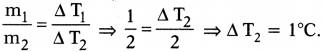So the rise in temperature for 2 kg of water = 1°C.Question 18.
What role does specific heat play in keeping a watermelon cool for a long time after removing it from a fridge on a hot day? (AS7)

• Generally, watermelon contains large percentage of water.
• Water has high specific heat value than other substances.
• High specific heat substances oppose the increase of temperature. Hence they continuous of the coolingness.
• So watermelon retains coolness after removing from fridge on a hot day due to the high specific cheat of water.

Question 19.
If you are chilly outside the shower stall, why do you feel warm after the bath if you stay in bathroom? (AS7)

• In the bathroom, the number of vapour molecules per unit volume is greater than the number of vapour molecules per unit volume outside the room.
• When we try to dry ourselves with a towel, the vapour molecules surrounding you condense on your skin.
• Condensation is a warming process.
• Because of the condensation, you feel warm outside the shower stall when it is chilly.

Question 20.
Three objects A at 30°C, B at 303K and C at 420 K are in thermal contact. Then answer the follwing questions.
(i) Which are in “Thermal equibrium” among A, B and C?
(ii) From which object to another heat transferred? (2 Marks)
i) 303K – 273K + 30K = 0°C + 30°C = 30°C.
∴ A and B objects are in ‘Thermal equibrium”.
ii) From object ‘C’ to objects ‘A’ and ‘B’ heat transferred.

Fill in the Blanks

1. The SI unit of specific heat is …………………. .
2. …………………. flows from a body at higher temperature to a body at lower temperature.
3. …………………. is a cooling process.
4. An object A at 10° C and another object B at 10 K are kept in contact, then heat will flow from …………………. to …………………. .
5. The latent heat of fusion of ice is …………………. .
6. Temperature of a body is directly proportional to …………………. .
7. According to the principle of method of mixtures, the net heat lost by the hot bodies is equal to …………………. by the cold bodies.
8. The sultryness in summer days is due to
9. …………………. is used as a coolant.
10. Ice floats on water because …………………. .

1. J/kg – K
2. Heat
3. Evaporation
4. A, B
5. 80 cal/gm
6. Average kinetic energy of the molecules of the body.
7. net heat gained
8. high humidity
9. Water
10. the density of ice is less than that of water

Multiple Choice Questions

1. Which of the following is a warming process?
A) evaporation
B) condensation
C) boiling
D) all the above
B) condensation2. Melting is a process in which solid phase changes to ………………. .
A) liquid phase
B) liquid phase at constant temperature
C) gaseous phase
D) any phase
B) liquid phase at constant temperature

3. Three bodies A, B and C are in thermal equilibrium. The temperature of B is 45° C. Then the temperature of C is ……………… .
A) 45° C
B) 50° C
C) 40° C
D) any temperature
A) 45° C

4. The temperature of a steel rod is 330 K. Its temperature in ° C is ……………… .
A) 55° C
B) 57° C
C) 59° C
D) 53° C
B) 57° C

5. Specific heat S =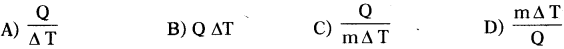6. Boiling point of water at normal atmospheric pressure is ……………… .
A) 0° C
B) 100° C
C) 110° C
D) -5° C
B) 100° C7. When ice melts, its temperature ……………… .
A) remains constant
B) increases
C) decreases
D) cannot say
A) remains constant

### 10th Class Physics 1st Lesson Heat InText Questions and Answers

10th Class Physics Textbook Page No. 1

Question 1.
Take a piece of wood and a piece of metal and keep them in a fridge or ice box. After 15 minutes, take them out and ask your friend to touch them. Which is colder? Why?
1) The metal piece is colder than the wooden piece.
2) Because more heat energy flows out of our body so metal piece gives coldness to our body, than wooden piece.

Question 2.
What could be the reason for difference in coldness of metal and wood?

• Due to more heat energy loss by our body when touches the metal piece compared to the wooden piece.
• In other way we say degree of coldness of the metal piece is greater than that of. the wooden piece.Question 3.
Does it have any relation to the transfer of heat energy from our body to the object?

• Yes, the principle of calorimetry, means heat loss by hot body is equal to heat gained by cold body.
• This means that when heat energy flows out of our body we feel the coldness and when heat energy enters our body we feel hotness.

10th Class Physics Textbook Page No. 2

Question 4.
Why does transfer of heat energy take place between objects?

• Due to the temperature difference between the two bodies which are in thermal contact.
• Now heat energy transfers from hot body to cold body until they attain same temperature.

Question 5.
Does transfer of heat take place in all situations?
No, when the bodies are in thermal equilibrium there is no transfer of heat energy.

Question 6.
What are the conditions for transfer of heat energy?

• Two bodies should have difference in temperature.
• They (two bodies) are in thermal contact with each other.
• When the bodies have equal temperature there is no transfer of heat energy.Question 7.
What is temperature?
(OR)
Define temperature.
Temperature : The measure of hotness or coldness of a body is called temperature.

Question 8.
How can you differentiate temperature from heat?

• Heat is a thermal energy that flows from hot body to cold body. Temperature is measure of the hotness or coldness of a body.
• Temperature decides direction of heat (energy) flow, whereas heat is energy itself that flows.

10th Class Physics Textbook Page No. 2 & 3

Question 9.
Place a laboratory thermometer in a glass tumbler containing hot water. Observe the change in mercury level. Wffet change did you notice in mercury level? Did mercury level increase or decrease?
The mercury level rises up that means temperature of the mercury level increases.

10th Class Physics Textbook Page No. 3

Question 10.
Place a laboratory thermometer in a glass tumbler containing cold water. Observe the change in mercury level. Did mercury level decrease or increase?
The mercury level falls down that shows temperature of the mercury level decreases.Question 11.
If two different systems A and B in thermal contact, are in thermal equilibrium individually with another system C (thermal contact with A and B), will the systems A and B be in thermal equilibrium with each other?
Yes, A and B will be in thermal equilibrium with each other that means A and B will have equal temperatures.

Question 12.
How would you convert degree Celsius to Kelvin?
Temperature in Kelvin = 273 + Temperature in degree Celsius. [K = t°C + 273]

10th Class Physics Textbook Page No. 4

Question 13.
Take two bowls one with hot water and second with cold water. Gently sprinkle food colour on the surface of the water in both bowls. How do food grains move? Why do they move randomly?
We will notice that the grains of food colour move randomly (jiggle). This happens because of the molecules of water on both bowls are in random motion.Question 14.
Why do the grains in hot water move more rapidly than the grains in cold water?

• Temperature kinetic energy. So molecules in hot water have more KE than molecules in cold water.
• As water molecules in hot water move rapidly, grains in hot water move more rapidly than the grains in cold water.

10th Class Physics Textbook Page No. 4 & 5

Question 15.
a) Take a cylindrical jar and pour hot water and then coconut oil in the vessel (do not mix them). Keep thermometers in hot water and coconut oil as shown in figure. The reading of thermometer in hot water decreases, at the same time reading of the thermometer, kept in oil increases. Why does this happen?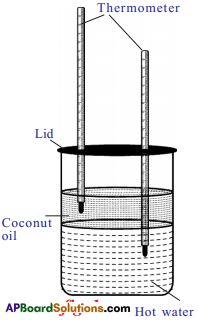• Heat transfers from hot water to oil.
• So, water loses heat and shows downfall in temperature.
• Oil takes the heat and shows increasing in the temperature.

b) Can you say that water loses energy’?

• Yes. Due to the temperature difference between the water and oil, water loses energy and oil gains energy.
• Thus some heat energy flows from water to oil.
• This means, the kinetic energy of the molecules of water decreases while the kinetic energy of molecules of oil increases.

c) Can you differentiate between heat and temperature based on the heat transmit activity?
Heat is the energy that flows from a hotter body to a colder body. Temperature denotes which body is hotter and which is colder. So, temperature determines direction of heat (energy) flow, whereas heat is the energy that flows.

10th Class Physics Textbook Page No. 5 & 6

Question 16.
Place two test tubes containing 50 gm of water, 50 gm of oil in boiling water for same time.
a) In which material does the temperature rise quickly? Are the amounts of heat given to the water and oil same? How can you assume this?

• Rise in temperature of oil is faster than the water.
• Yes, same amount of heat energy given to both the oil and water through boiling water.b) Why does this happen in specific heat?
This happens because rise in temperature depends on the nature of substance.

10th Class Physics Textbook Page No. 7

Question 17.
How much heat energy is required to rise the temperature of unit mass of substance (material) by 1°C?
Energy equal to its specific heat.Question 18.
Why is the specific heat different for different substances?
(OR)
Explain why specific heat values are different for different materials.

• We know that the temperature of the body is directly proportional to the average kinetic energy of particle of the body.
• The molecules of the system have different forms of energies such as linear, rotational kinetic energy, vibrational energy and potential energy.
• When we supply heat energy, it will be shared in different forms and increase the energy in the system.
• This sharing will vary from material to material.
• If the maximum share of heat energy is spent to rise linear kinetic energy, then the system gets increasing in temperature.
• Due to differences in sharing different materials have different specific heats.

10th Class Physics Textbook Page No. 8

Question 19.
Take 200 ml of water in two beakers and heat them to same temperature and pour the water of two beakers into a larger beaker.
What do you observe? What could be the reason for the fact you observed?

• The temperature of mixture remains the same.
• The reason is that the masses rise in temperature and the materials are same.

Question 20.
Heat the water in one beaker to 90°C and the other to 60°C. Mix the water from these beakers in large beaker. What will be the temperature of the mixture? What did you notice? Can you give reason for the change in temperature?

• The temperature of mixture is 75°C.
• The reason is for a given material the temperature of mixture,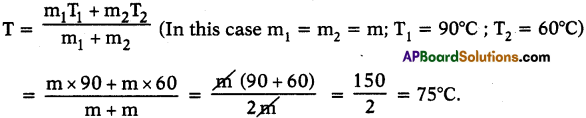• Hot water gives heat to the cold water until thermal equilibrium takes place.
• So, the thermal equilibrium attains at 75°C.

Question 21.
ake 100 ml of water at 90°C and 200 ml of water at 60°C and mix the two. What is the temperature of the mixture? What difference do you notice in change of temperature?

• The temperature of mixture is 70°C.
• The reason is here m1 = 100 gm ; m2 = 200 gm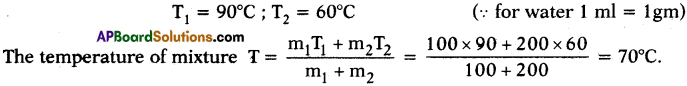• Final temperature of the mixture is less than the above case.
• If the quantity increases, the quantity of heat to transfer is also rises to attain thermal equilibrium.
Here hotter body quantity is less and colder body quantity is high. So, the temperature at thermal equilibrium decreases and stands at 70° C.

10th Class Physics Textbook Page No. 10

Question 22.
When floor of room is washed with water, the water on the floor disappears within minutes. Why does water on the floor disappear after some time?
Due to evaporation water disappears from the floor.

Question 23.
Pour a few drops of spirit on your palm. Why does your skin become colder? (1 Mark)
Spirit absorbs heat energy from our palm and evaporates. So our palm becomes colder.

10th Class Physics Textbook Page No. 10 & 11

Question 24.
Take a few drops of spirit in two petri dishes separately. Keep one of the dishes under a ceiling fan and switch on the fan. Keep another dish with its lid closed. What do you notice? What could be the reason for this change?
1) The spirit in the dish which is kept under the ceiling fan disappears.
2) Whereas we will find some spirit left in the dish that is kept in the lidded dish.
3) The molecules which are escaping from the surface is high and they can’t reach back to liquid due to wind blow. So, evaporation is high under fan.
4) At the same time evaporation is less in the dish which is closed by lid.

10th Class Physics Textbook Page No. 12

Question 25.
Does the reverse process of evaporation take place? When and how does it take place?
1) Yes, the reverse process of evaporation takes place.
2) When the vapour molecules lose their kinetic energy which leads to lower the temperature, they convert into droplets.
3) This process is called condensation.

10th Class Physics Textbook Page No. 13

Question 26.
In early morning, during winter, you might have noticed that water droplets form on window panes, flowers, grass, etc. How are these water droplets formed?
(OR)
Why do water drops (dew) form on flowers and grass during morning hours of winter season?

• During winter season, in the night times, atmospheric temperature goes down.
• The surfaces of window panes, flowers, grass, etc. become colder.
• The water vapour molecules touch the surfaces, gets cooled and lost its energy.
• Then water vapour condenses on the surface and water drops formed.
• The water droplets condensed on such surfaces are known as dew.

10th Class Physics Textbook Page No. 14

Question 27.
Are the process of evaporation and boiling the same? Explain.

• No, they are different.
• Evaporation takes place at any temperature.
• But boiling occurs at particular temperature called the boiling point.

10th Class Physics Textbook Page No. 16

Question 28.
You might have observed coconut oil and ghee getting converted from liquid state to solid state during winter season. What could be the reason for this change? What happens to water kept in a refrigerator? How does it get converted from liquid phase to solid phase?
1) If temperature of a substance decreases kinetic energy also decreases.
2) Kinetic energy decreases from water to ice. That means solid state to liquid state.
3) In winter season coconut oil in the form of liquid get down its temperature, hence its kinetic energy also decreases. So, it-freezes.
4) Water, which is kept in refrigerator loses the kinetic energy along with decreasing temperature and freezes.
5) In this way water converted liquid phase to solid phase.Question 29.
Are the volumes of water and ice formed with same amount of water equal? Why?
1) No, the volume of ice is greater than volume of water.
2) Water expands on freezing.
3) That means density of ice is less than density of water.

10th Class Physics 1st Lesson Heat Activities

Activity – 1

1. Explain the term temperature with example.
(OR)
What is the name given to degree of hotness or coldness? Explain the quantity with an example.
Procedure: Take a piece of wood and a piece of metal and keep them in fridge or ice box.
Observation : When we touch both of them we feel that metal piece is colder than the wooden piece.

Explanation :

• This is due to more energy flow out of our body when we touch the metal piece as compared with wooden piece.
• The degree of coldness of metal is greater than that of the wooden piece.
• The degree of hotness or coldness is called temperature.
• From this example, we say metal piece is at a lower temperature compared to wooden piece.

Activity – 2

2. What is the measure of thermal equilibrium? How do you prove?
(OR)
How do you prove temperature is the measure of thermal equilibrium?
(OR)
Explain thermal equilibrium with an activity.
Procedure :
Take two glass tumblers and fill one of them with hot water and another with cold water.
Explanation & Observation :
1) When we place a thermometer inside the hot water the mercury level of thermometer rises from initial position due to heat transferred from hotter body (hot water) to colder body (mercury in thermometer).
2) When we place the thermometer inside the cold water the mercury level comes down from its initial position due to transfer of heat from mercury (hotter body) to water (colder body).

Conclusion :

• Heat is a form of energy that flows from a body at higher temperature to a body at lower temperature until the temperature remains same for two bodies that is called thermal equilibrium.
• In the above case, the steadiness of mercury column shows that thermal equilibrium is achieved. That reading of mercury column gives temperature.
• Thus temperature is a measure of thermal equilibrium.

Activity – 3

3. Establish the relationship between temperature and average kinetic energy.
(OR)
Suggest an activity to prove that the average kinetic energy of the molecules is directly proportional to the absolute temperature of the substances.
(OR)
How do you prove that temperature of a body is an indicator of average kinetic energy?
Procedure :

• Take two bowls one with hot water and second with cold water.
• Gently sprinkle food colour on the surface of the water in both bowls.

Observation :
We will observe the jiggling of grains of food colour in hot water is more when compared to jiggling in cold water.

Explanation :

• We know kinetic energy depends on speed motion of particles.
• So the kinetic energy of hotter body is greater than that of colder body.
• Thus the temperature of a body is an indicator of average kinetic energy of molecules of that body.

Conclusion :
Therefore average kinetic energy of molecules is directly proportional to absolute temperature.

Activity – 4

4. Write an activity which tells how heat transmits.
(OR)
In which direction does heat tend to flow? Prove it with an activity.Procedure :

• Take water in a container and heat it to 60° C.
• Take a cylindrical transparent glass jar and fill half of it with the hot water.
• Pour coconut oil over the surface of water.
• Put a lid with two holes on the top of the glass jar.
• Insert two thermometers through the lid in such a way that one inside coconut oil and other in water.

Observation & Explanation :

• Now we can observe that the reading of thermometer kept in water decreases while the reading of thermometer kept in oil increases.
• So temperature of water decreases whereas temperature of oil increases.

Conclusion :

• Heat transmits from hotter body to colder body.
• So temperature determines direction of heat flow.

Activity – 5 Specific Heat

5. Write an activity which gives the relation between rise in temperature and nature of material.
(OR)
“The rate of rise in temperature depends on the nature of substance.” Prove it with an activity.
(OR)
Draw a diagram and label the parts to prove that the rate of increase in temperature depends on the nature of substance.
(OR)
We can observe severe burns with hot oil when compared with hot water.
Which factor will decide this aspect? Explain this process with an example.
Procedure :

• Take a large jar with water and heat it up to 80°C.
• Take two identical boiling test tubes with single-holed corks.
• Fill them, one of the boiling tubes with 50 gm of water and other with 50 gm of oil.
• Insert two thermometers in each of tubes and clamp them to retort stand and place them in a jar of hot water.

Observation :

• Observe the readings of thermometers every three minutes.
• We can observe that the rise in temperature of oil is higher than that of water.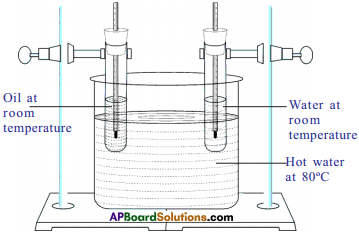Explanation :

• Since both the boiling tubes kept in hot water for the same interval of time, the heat supplied to oil and water is same but rise in temperature of oil is more.
• So we conclude that rise in temperature depends on the nature of substance (specific heat).

Activity – 6

6. Derive Q = mSAT.
(OR)
Establish relationship between heat energy, mass of the substance and rise in temperature.
(OR)
Derive an expression for heat energy.
(OR)
Derive an expression for factors affecting amount of heat energy absorbed.
Procedure :

• Take two beakers of equal volume and take 250 grams of water in one beaker and 1 kg of water in another beaker.
• Note their initial temperatures.
• Now heat the two beakers up to 60° C.
• Note down the heating times.

Observation:

• We observe that-the water in large beaker takes more time.
• That means we need to supply more heat enepgy to water in larger beaker (greater quantity of water).

Conclusion :
From this we conclude that for some change in temperature the amount of heat (Q) absorbed by a substance is directly proportional to its mass (m).
Q ∝ m (when ∆T is constant) ………….. (1)

Procedure :
Now take 1 litre of water in a beaker and heat it and note the temperature changes (∆T) for every two minutes and observe the rise internals.

Conclusion:
We will notice that for the same mass (m) of water the change in temperature is proportional to amount of heat (Q) absorbed by it.
Q ∝ ∆T (when m is constant) ………….. (2)
From (1) and (2) Q m∆T (or) Q = mS∆T,
where ‘S’ is called specific heat of substance.

Activity – 7

7. a) How are you able to find the final temperature of the mixture of sample?
(OR)
What is the “Principle of method of mixtures”? Verify it with an activity.
Situation – 1 :

• Take two beakers of the same size and pour 200 ml of water in each of them.
• Now heat the water in both beakers to same temperature.
• Now pour water from these beakers into a larger beaker and measure the temperature of the mixture.

Observation :
We can observe that there is no change in temperature.

Situation – 2 :

• Now heat the water in first beaker to 90° C and the other to 60° C.
• Mix the water from these beakers in a large beaker.

Observation :
We can find that the temperature of mixture is 75° C.

Situation – 3 :
Now take 100 ml of water at 90° C and 200 ml of water at 60° C and mix the two. Observation :
We can find that the temperature of mixture is 75° C.7. b) Derive, a formula for final temperature of mixture of samples.
(OR)
Maveen added hotter water of mass m1 kept at temperature T1 to cold water of mass m2 kept at temperature T2. Find the expression to find temperature of mixture of samples.
Procedure:
1) Let the initial temperatures of the hotter and colder samples of masses m1 and m2 be T1 and T2.
2) Let T be the final temperature of mixture.

Observation :
The temperature of the mixture is lower than hotter sample and higher than colder sample. Explanation :
So hot sample has lost heat, and the cold sample has gained heat.
The heat lost by the hot sample Q1 = m1S (T1 – T)
The heat gained by the cold sample Q2 = m2S (T – T2)
We know that heat lost = heat gained
Q1 = Q2
m1 S(T1 – T) = m2 S(T – T2)
$$\mathrm{T}=\frac{\mathrm{m}_{1} \mathrm{~T}_{1}+\mathrm{m}_{2} \mathrm{~T}_{2}}{\mathrm{~m}_{1}+\mathrm{m}_{2}}$$

Activity -8

8. Explain the process of evaporation phenomenon with an example.
(OR)
Srinu observed that spirit taken in a petri dish disappears after some time. Explain the process involved in it with an example.
Procedure :

• Take a few drops of spirit in two petri dishes separately.
• Keep one of the dishes under a ceiling fan and keep another dish with its lid closed.
• Observe the quantity of spirit in both dishes after 5 minutes.

Observation :
We will notice that spirit in the dish kept under the ceiling fan disappears whereas we will find some spirit left in the dish that is kept in the lidded dish.

Explanation :

• The reason is that the molecules of spirit in dish continuously move with random speeds and collide with other molecules.
• During the collision they transfer energy to other molecules.
• Due to this collision the molecules at the surface acquire energy and fly off from the surface.
• Some molecules come back to liquid.
• If the number of escaping molecules is greater than returned number, then the number of molecules in the liquid decreases.

Conclusion :
When a liquid is exposed to air, the molecules at the surface keep on escaping from the surface till the entire liquid disappears into air. This process is called evaporation.

Activity – 9

9. Explain the process of condensation with example.
(OR)
Explain the process of condensation with an activity.
(OR)
Karan told his friend that he observed that there are some water droplets outside a cold soft drink bottle. Explain the phenomenon involved in the formation of these droplets.
Procedure :
Place a glass tumbler on the table. Pour cold water up to half of its height.

Observation :
There are droplets formed outside of the glass.

Explanation :

• The reason is that the surrounding air contains water molecules in the form of water vapour. When the water molecules strike the surface of the glass tumbler which is cool, they lose their kinetic energy.
• This energy lowers the temperature of vapour and it turns into droplets.
• The energy lost by water molecules in air is gained by the molecules of the glass tumbler.
• Hence the average kinetic energy of glass molecules increases. In turn the energy is transferred to water molecules in die glass.

Conclusion :

• So the average kinetic energy and temperature of water in glass increases. This is called condensation.
• Condensation is the phase change from gas to liquid.

Activity – 10

10. Explain the process of boiling with an example.
(OR)
Why do we observe bubbles on the surface of water which has been heated ? What is the phenomenon involved in it? Explain.
Procedure :
Take a beaker of water, keep it on the burner and note the readings of thermometer for every two minutes.

Observation :

• We will notice that the temperature of the water rises continuously till it reaches 100° C.
• Once it reaches 100° C the temperature remains same and a lot of bubbling on the surface takes place. This is called boiling of water.

Explanation :

• It happens due to when water is heated the solubility of gases it contains reduces.
• As a result, bubbles of gas are formed in the liquid.
• Evaporation of water molecules from the surrounding liquid occurs into these bubbles and they become filled with saturated vapour.
• At a certain temperature, the pressure of the saturated vapour inside the bubbles becomes equal to the pressure exerted on the bubbles from the outside.

Conclusion :

• As a result, these bubbles rise rapidly to the surface and collapse at the surface releasing vapour present in bubbles into air at the surface. This process is called “boiling”.
• This temperature is called ‘boiling temperature”.

Activity – 11

11. Explain the process of melting and latent heat of fusion.
(OR)
When ice is heated to 0°C it starts to turn into water. But temperature remains ‘ constant for some time. What is the process involved in this? Explain.
Procedure :

• Take small ice cubes in a beaker. Insert the thermometer in the beaker.
• Now start heating the beaker and note down readings of thermometer every one minute till the ice completely melts and gets converted into water.
• Before heating the temperature of ice is 0°C or less than 0°C.

Observation :

• We will observe that the temperature of ice at the beginning is equal to or below 0°C.
• If the temperature of ice is below 0°C, it goes on changing till it reaches 0°C.
• When ice starts melting, we will observe no change in temperature though you are supplying heat continuously.

Explanation:

• Given heat energy uses to break the bonds (H2O) in ice and melts.
• So, temperature is constant while melting.

Conclusion:

• This process is called melting. In this process, heat converts solid phase to liquid phase.
• The temperature of the substance does not change until all the ice melts and converts into water.
• The heat given to melting is called latent heat of fusion.
• The heat required to convert 1 gm of solid completely into liquid at constant temperature is called “latent heat of fusion”.

Activity – 12

12. Why does a glass bottle tilled with water break when it is placed in deep freezer for some time?
(OR)
Prove that density of ice is less than that of water.
(OR)
How do you prove that volume of ice is more than that of water?
Procedure :

• Take a small glass bottle with a tight lid and fill it with water, without any gap and fix the lid tightly.
• Put the bottle into the deep freezer of a refrigerator for a few hours.

Observation :
When we take it out from the deep freezer, we can observe that the glass bottle breaks.

Explanation :

• These cracks on the bottle due to expansion of the substance in the bottle.
• This means water expands on freezing.

Conclusion :

• Water expands on freezing.
• Ice has less density than water.